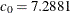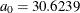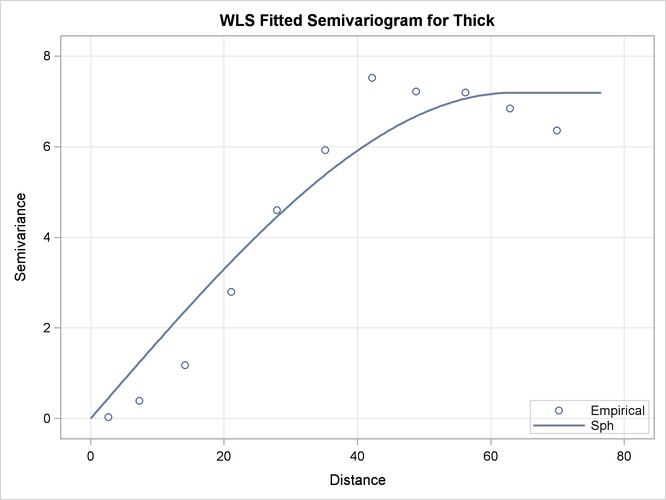### Example 49.2 Investigating the Effect of Model Specification on Spatial Prediction

It is generally believed that spatial prediction is robust against model specification, while the standard error computation is not so robust. This example investigates the effect of using these different models on the prediction and associated standard errors.

In the section Theoretical Semivariogram Model Fitting in the VARIOGRAM procedure, a particular theoretical semivariogram is fitted to the coal seam thickness data empirical semivariogram. The chosen semivariogram is Gaussian with a scale (sill) ofand a range of.

Another possible model choice could be the spherical semivariogram. First, use a DATA step to input the thickness data:

```title 'Effect of Model Specification on Prediction';

data thick;
input East North Thick @@;
label Thick='Coal Seam Thickness';
datalines;
0.7  59.6  34.1   2.1  82.7  42.2   4.7  75.1  39.5
4.8  52.8  34.3   5.9  67.1  37.0   6.0  35.7  35.9
6.4  33.7  36.4   7.0  46.7  34.6   8.2  40.1  35.4
13.3   0.6  44.7  13.3  68.2  37.8  13.4  31.3  37.8
17.8   6.9  43.9  20.1  66.3  37.7  22.7  87.6  42.8
23.0  93.9  43.6  24.3  73.0  39.3  24.8  15.1  42.3
24.8  26.3  39.7  26.4  58.0  36.9  26.9  65.0  37.8
27.7  83.3  41.8  27.9  90.8  43.3  29.1  47.9  36.7
29.5  89.4  43.0  30.1   6.1  43.6  30.8  12.1  42.8
32.7  40.2  37.5  34.8   8.1  43.3  35.3  32.0  38.8
37.0  70.3  39.2  38.2  77.9  40.7  38.9  23.3  40.5
39.4  82.5  41.4  43.0   4.7  43.3  43.7   7.6  43.1
46.4  84.1  41.5  46.7  10.6  42.6  49.9  22.1  40.7
51.0  88.8  42.0  52.8  68.9  39.3  52.9  32.7  39.2
55.5  92.9  42.2  56.0   1.6  42.7  60.6  75.2  40.1
62.1  26.6  40.1  63.0  12.7  41.8  69.0  75.6  40.1
70.5  83.7  40.9  70.9  11.0  41.7  71.5  29.5  39.8
78.1  45.5  38.7  78.2   9.1  41.7  78.4  20.0  40.8
80.5  55.9  38.7  81.1  51.0  38.6  83.8   7.9  41.6
84.5  11.0  41.5  85.2  67.3  39.4  85.5  73.0  39.8
86.7  70.4  39.6  87.2  55.7  38.8  88.1   0.0  41.6
88.4  12.1  41.3  88.4  99.6  41.2  88.8  82.9  40.5
88.9   6.2  41.5  90.6   7.0  41.5  90.7  49.6  38.9
91.5  55.4  39.0  92.9  46.8  39.1  93.4  70.9  39.7
55.8  50.5  38.1  96.2  84.3  40.3  98.2  58.2  39.5
;
```

Fitting of the Gaussian model is performed in the section Theoretical Semivariogram Model Fitting in the VARIOGRAM procedure, and the fitting parameters are saved in the `SemivStoreGau` item store with the following statements:

```ods graphics on;
```
```proc variogram data=thick noprint;
store out=SemivStoreGau / label='Thickness Gaussian Model';
compute lagd=7 maxlag=10;
coord xc=East yc=North;
model form=gau;
var Thick;
run;
```

For prediction with the saved Gaussian model, you use the following statements to run the KRIGE2D procedure with input from the `SemivStoreGau` item store. You invoke the item store with the RESTORE statement. The STORESELECT option in the MODEL statement that specifies that you want to use the selected model in the item store as input for your prediction.

```proc krige2d data=thick outest=pred1 noprint;
restore in=SemivStoreGau;
coordinates xc=East yc=North;
predict var=Thick r=60;
model storeselect;
grid x=0 to 100 by 10 y=0 to 100 by 10;
run;
```

Then, you run the KRIGE2D procedure by using a spherical model. Start by using the VARIOGRAM procedure to fit a spherical model to the `thick` data set empirical semivariogram. You specify the STORE statement again in PROC VARIOGRAM to save the spherical model estimated parameters in an item store with the name `SemivStoreSph`. You use the following statements:

```proc variogram data=thick plots(only)=fit;
store out=SemivStoreSph / label='Thickness Sph Model';
compute lagd=7 maxlag=10;
coord xc=East yc=North;
model form=sph;
var Thick;
run;
```

The VARIOGRAM procedure fits the spherical model successfully, and the estimated parameters for this fit are shown in Output 49.2.1.

Output 49.2.1: Spherical Model Fitting Parameter Estimates

 Effect of Model Specification on Prediction

The VARIOGRAM Procedure
Dependent Variable: Thick
Angle: Omnidirectional
Current Model: Spherical

Parameter Estimates
Parameter Estimate Approx
Std Error
DF t Value Approx
Pr > |t|
Nugget 0 0 8 . .
Scale 7.1914 0.2827 8 25.44 <.0001
Range 63.2351 4.1050 8 15.40 <.0001

The fit summary is displayed in Output 49.2.2. When compared to the corresponding result in the section Theoretical Semivariogram Model Fitting in the VARIOGRAM procedure, the goodness-of-fit criteria indicate a worse statistical fit for the spherical model compared to the Gaussian.

Output 49.2.2: Spherical Model Fit Summary

Fit Summary
Model Weighted
SSE
AIC
Sph 52.26791 23.14336

Output 49.2.3 suggests an acceptable fit of the spherical model to the `thick` data set. Obviously, the fit of the spherical model in the sensitive area near the semivariogram origin is less faithful to the empirical semivariance than the Gaussian model. The following analysis explores the consequence in the kriging prediction of this discrepancy.

Output 49.2.3: Fitted Spherical and Empirical Thick SemivariogramFor the next step, you run the KRIGE2D procedure by using the spherical model parameters stored in the `SemivStoreSph` item store. You use the following statements:

```proc krige2d data=thick outest=pred2 noprint;
restore in=SemivStoreSph;
coordinates xc=East yc=North;
predict var=Thick r=60;
model storeselect;
grid x=0 to 100 by 10 y=0 to 100 by 10;
run;
```

Eventually, you compare the prediction results and errors of the two models. You use a DATA step to compute the relative difference of the predicted values and the prediction error for each one of the Gaussian and the spherical models. You store the prediction relative difference in the `prdRelDiff` variable and the prediction relative error in the `stdRelDiff` variable. You save the output in the `compare` data set with the following statements:

```data compare;
merge pred1(rename=(estimate=g_prd stderr=g_std))
pred2(rename=(estimate=s_prd stderr=s_std));
prdRelDif = ((g_prd-s_prd) / s_prd) * 100;
stdRelDif = ((g_std-s_std) / s_std) * 100;
run;
```

The MEANS procedure uses the `compare` data set to produce statistics about the prediction relative difference and error for each one of the `prdRelDiff` and `stdRelDiff` variables with the following statements:

```proc means data=compare;
var prdRelDif stdRelDif;
run;

ods graphics off;
```

Output 49.2.4 shows that on average the predicted values are very close for the two semivariogram models. The mean relative difference in the prediction values is close to zero with a low standard deviation, whereas the relative difference values fluctuate with an absolute maximum of about 5%.

However, note that the mean relative standard error is about -96%. According to the definition of the `stdRelDiff` variable, the high negative value indicates that the prediction error difference between the two models is very close to the spherical model prediction error. Hence, the prediction standard error of the spherical model is substantially larger than that of the Gaussian model. In fact, the prediction relative error never gets smaller than about 66% for the two models, where the negative sign in the `Minimum` and `Maximum` columns in Output 49.2.4 means that the prediction error is always greater for the spherical model.

Output 49.2.4: Comparison of Gaussian and Spherical Models

 Effect of Model Specification on Prediction

The MEANS Procedure

Variable N Mean Std Dev Minimum Maximum
prdRelDif
stdRelDif
 121 121
 -0.0544593 -96.2515
 1.3384 5.94
 -5.07514 -99.8974
 5.19262 -65.9276